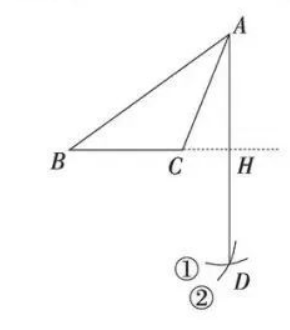$\text{A.}$ $B H$ 垂直平分线段 $A D$ $\text{B.}$ $A C$ 平分 $\angle B A D$ $\text{C.}$ $S_{\triangle A C}=B C \cdot A H$ $\text{D.}$ $A B=A D$